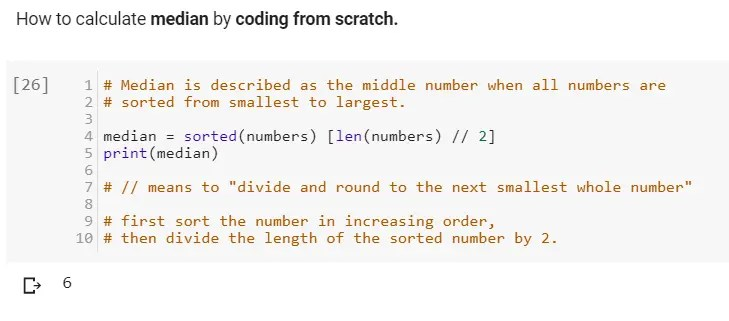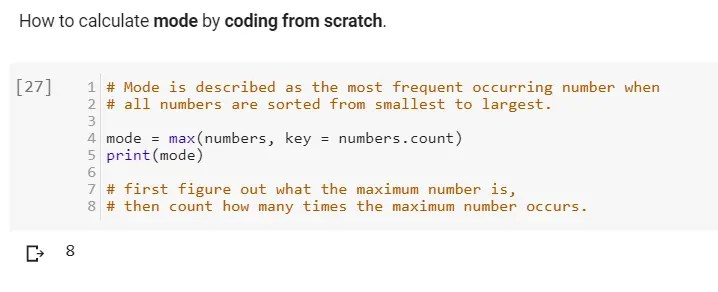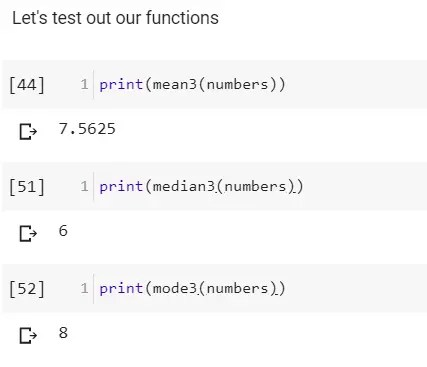# 3 ways to calculate Mean, Median, and Mode in Python

In this content, we are going to cover how to Calculate Mean, Median, and Mode in Python (3 ways)

1. How to calculate mean, median, and mode in python by coding it from scratch.
2. How to calculate mean, median, and mode in python by using python libraries.
3. How to calculate mean, median, and mode in python by creating python functions.

LET’S DIVE IN…

Before we get into how to write these calculations by code, lets first define what is mean, median, and mode in mathematical terms.

Mean is described as the total sum of the numbers in a list divided by the length of the numbers in the list. In simple translation, take all the number in a list and add them up, then divided the total by how many numbers are on the list.

Median is described as the middle number when all numbers are sorted from smallest to largest. In simple translation, sort all numbers in a list from the smallest one to the largest one. Whichever number is in the middle is the median. In the case of having two numbers in the middle, add the two middle numbers together and divide them by 2. The resulting answer from the division by 2 is the median.

Mode is described as the most frequent occurring number when all numbers are sorted from smallest to largest. In simple translation, when you have a list of numbers, which ever number occurs more often than the other numbers is the mode. If all numbers occurs the same amount of time, then the set of numbers has no mode.

Now  that we have basic definition and understanding of mean, median, and mode, lets go ahead and set up the python calculations.

### GRAB MY FREE DATA SCIENCE RESOURCES

1. How to calculate mean, median, and mode in python by coding it from scratch.

We will start by learning how to compute mean, median, and mode from scratch without any added help.How to calculate mean in python by coding from scratch manually.How to calculate median in python by coding from scratch manually.How to calculate mode in python by coding from scratch manually.

2. How to calculate mean, median, and mode in python by using python libraries and modules.

Now we are going to use a python module to calculate mean, median and mode.

### GRAB MY FREE DATA SCIENCE RESOURCES

3. How to calculate mean, median, and mode in python by creating python functions.

Up next, we will be writing a function to compute mean, median, and mode in python.

Why Function?

To make calculating mean, median, and mode easy, you can quickly write a function that calculates mean, median, and mode.

The purpose of this function is to save you time and energy of typing in the mean, median, and mode formula repeatedly to do calculations through out your code.

Whenever you want to do mean, median, and mode calculation, you can just call the mean, median, and mode function instead of typing the formula code again.

If we test our function above with the same “numbers” we have been using, these are the results.The results for using python function to calculate mean, median, and mode.

I hope you enjoyed this content on 3 ways to calculate mean, median, and mode in python.

Leave a comment below to let me know other ways to calculate mean, median, and mode in python and what you think of this tutorial

### GRAB MY FREE DATA SCIENCE RESOURCES

•find mode without using libraries

•if there are 2 modes it gives error so can you please post one with multiple mode

•If there are 2 modes, it’s called bimodal and 3 modes is called trimodal. just pick one as your mode if you must pick one or just report all your modes. I hope this helps 😏😊

•This is most informative and also this post most user friendly and super navigation to all posts… Thank you so much for giving this information to me..

•Hi ,
there are many other statistical functions so can you brief them using normal python code but not by using in-build methods.
And can you let me know how to produce output using the below format as each and every number needs to be allocated a memory
a=[1,2,3,——10]
mean(a)=
mean(a)=

co-variance(a)
co-relation(a)
cpk and cp…..etc..,

••